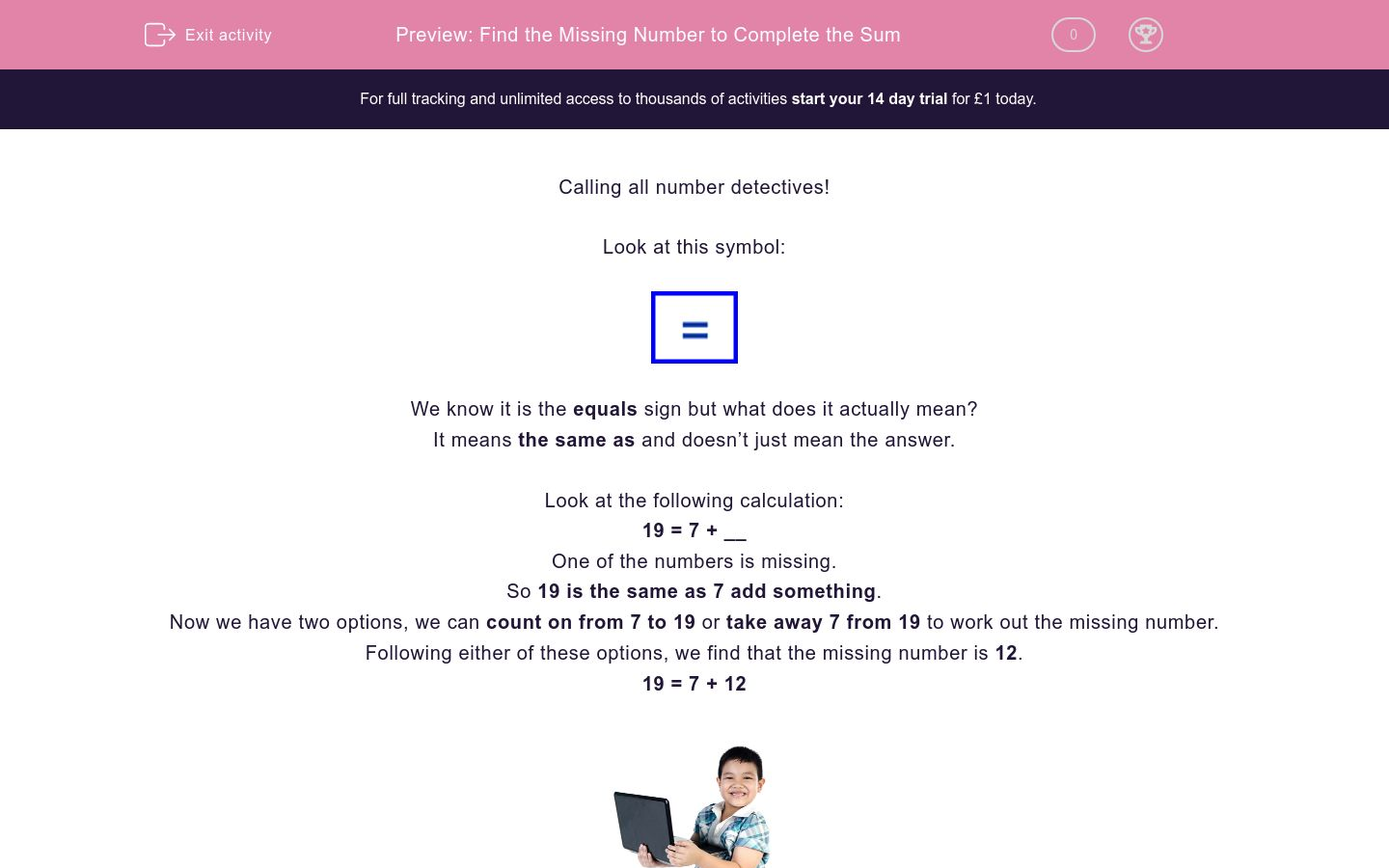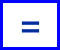Find the Missing Number to Complete the Sum

In this worksheet, students will complete a calculation by adding in the missing numbers. It will strengthen their number skills.Key stage:  KS 2

Curriculum topic:  Verbal Reasoning

Curriculum subtopic:  Complete the Calculation

Difficulty level:QUESTION 1 of 10

Calling all number detectives!

Look at this symbol:We know it is the equals sign but what does it actually mean?

It means the same as and doesn’t just mean the answer.

Look at the following calculation:

19 = 7 + __

One of the numbers is missing.

So 19 is the same as 7 add something.

Now we have two options, we can count on from 7 to 19 or take away 7 from 19 to work out the missing number.

Following either of these options, we find that the missing number is 12.

19 = 7 + 12Let’s try another:

17 = 20 -__

In this question, the answer of 17 is the same as 20 take away something.

What do we need to take away from 20 to reach 17?

So the missing number is 3.

17 = 20 - 3

Pssstt!! Here’s a handy hint to help you reach superstar status:

Remember that equals means the same as and the answers on either side of the equals sign must be the same.

In this activity, you will be completing sums by finding the missing number.

Good luck!

Welcome number detective!

Below is a calculation. One of the numbers has been taken away by the cheeky number burglar!

Can you work out what it is number detective?

__ = 12 + 11Shall we try another one?

Below is a calculation. One of the numbers has been taken away by the cheeky number burglar!

Can you work out what it is,  number detective?

__ = 13 + 13Below is another calculation. As before, one of the numbers has been taken away by the cheeky number burglar!

Can you work out what this one is number detective?

__ = 19 + 11I think you've probably got the idea by now!

Below is a calculation. One of the numbers has been taken away by the cheeky number burglar!

Can you use your super detecting skills to work out what it is?

__ = 28 - 12Halfway there already, number detective!

Below is another calculation. One of the numbers has been taken away by that really irritating number burglar!

Can you work out what it is?

__ = 11 x 2How are you getting on with these number detective? You're getting through these activities really quickly, so don't give up!

Below is a calculation. That wretched number burglar has done it again and  taken away one of the numbers! Can you work out what it is?

__ = 6 x 5Here we go again!

Below is a calculation. One of the numbers is missing - could that number burglar be up to his old tricks again?

Can you work out what number it is, number detective?

__ = 29 - 19Keep going, number detective - you're nearly there!

What number has that number burglar pinched this time?

__ = 12 x 10Below is another calculation.

Can you work out what number is missing?

__ = 9 x 2Here's the last activity.

Can you work out what number is missing from this calculation, number detective?

__ = 22 + 15• Question 1

Welcome number detective!

Below is a calculation. One of the numbers has been taken away by the cheeky number burglar!

Can you work out what it is number detective?

__ = 12 + 1123
EDDIE SAYS
The equals sign is at the start of the calculation, not the end, as it usually is, so we need to remember that this sign means 'the same as'. So, what could we put before the equals sign that is the same as the numbers 12 and 11 added together? The answer can be found by a simple addition sum: 12 + 11 which makes 23. How did you get on with this one number detective?
• Question 2

Shall we try another one?

Below is a calculation. One of the numbers has been taken away by the cheeky number burglar!

Can you work out what it is,  number detective?

__ = 13 + 1326
EDDIE SAYS
How did you find this one? Remember that the equals sign means 'the same as', so we need to add the two numbers together to get the answer: 13 + 13 gives us 26. If you are finding it tricky to work the answer out mentally, you may find a sheet of paper and pencil handy to do your working out on.
• Question 3

Below is another calculation. As before, one of the numbers has been taken away by the cheeky number burglar!

Can you work out what this one is number detective?

__ = 19 + 1130
EDDIE SAYS
Did you work out the answer number detective? Once again, you need to remember that the equals sign means 'the same as', so we need to add the two numbers together. Don't be put off by the fact that the answer to this addition calculation is on the left of the equals sign instead of the right, as it normally is. So, we get: 19 + 11 will give us the answer 30.
• Question 4

I think you've probably got the idea by now!

Below is a calculation. One of the numbers has been taken away by the cheeky number burglar!

Can you use your super detecting skills to work out what it is?

__ = 28 - 1216
EDDIE SAYS
A subtraction calculation this time. What method did you use to work it out? Did you count on from 12 to 28 to find what needs to be added to 12 to make the number 28? Or did you choose to subtract 12 from 28? You could try doing this mentally or use a written method to work it out as a column sum with the numbers written above each other. Whichever way you chose to use, you should have ended up with the answer 16.
• Question 5

Halfway there already, number detective!

Below is another calculation. One of the numbers has been taken away by that really irritating number burglar!

Can you work out what it is?

__ = 11 x 222
EDDIE SAYS
A different sort of sum again here, number detective! Remember that the equals sign means 'the same as' so you simply need to do the sum on the right hand side of the sign and put the answer on the left hand side. Did you work out that this uses your two times table? The calculation is: 11 x 2 and the answer is 22.
• Question 6

How are you getting on with these number detective? You're getting through these activities really quickly, so don't give up!

Below is a calculation. That wretched number burglar has done it again and  taken away one of the numbers! Can you work out what it is?

__ = 6 x 530
EDDIE SAYS
Are you getting the hang of these yet? If you are finding them difficult, just remember that the equals sign means 'the same as' and it doesn't matter which side of the sign the answer needs to be written. This question is testing your five times table knowledge - did you spot that? So, the calculation is 6 x 5 and the answer is 30. 30 = 6 x 5
• Question 7

Here we go again!

Below is a calculation. One of the numbers is missing - could that number burglar be up to his old tricks again?

Can you work out what number it is, number detective?

__ = 29 - 1910
EDDIE SAYS
OK, number detective, let's have a look at this one. This time you are being asked to find what is 'the same as' 29 - 19. So, it is a simple subtraction calculation. which can be worked out in several ways. One way to do this sum in your head would be to first subtract 10 from 29 to get 19 (29 - 10 = 19) and then subtract 9 from 19 to get the answer 10 (19 - 9 = 10). What method did you use?
• Question 8

Keep going, number detective - you're nearly there!

What number has that number burglar pinched this time?

__ = 12 x 10120
EDDIE SAYS
Once again, number detective, you need to remember that the equals sign means 'the same as'. So, all we have to do is find out what is the same as 12 x 10 so it is a ten times table calculation! Did you work out the answer? 120 is the same as 12 x 10. 120 = 12 x 10.
• Question 9

Below is another calculation.

Can you work out what number is missing?

__ = 9 x 218
EDDIE SAYS
How did you do with this one? Remember, it simply means what is the same as 9 x 2. So, this is a two times table question. The answer is therefore: 18 = 9 x 2. Let's try another one.
• Question 10

Here's the last activity.

Can you work out what number is missing from this calculation, number detective?

__ = 22 + 1537
EDDIE SAYS
Remember, that you have to find what 'is the same as' 22 + 15. Did you find the total of the two numbers? The answer is 37: 37 = 22 + 15. Thanks for your help number detective! There are still lots more numbers missing, so I hope to see you again soon for another amazing mission!
---- OR ----

Sign up for a £1 trial so you can track and measure your child's progress on this activity.

What is EdPlace?

We're your National Curriculum aligned online education content provider helping each child succeed in English, maths and science from year 1 to GCSE. With an EdPlace account you’ll be able to track and measure progress, helping each child achieve their best. We build confidence and attainment by personalising each child’s learning at a level that suits them.

Get startedStart your £1 trial today.
Subscribe from £10/month.